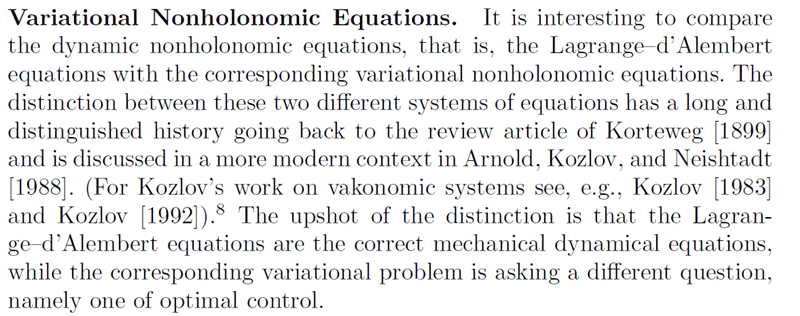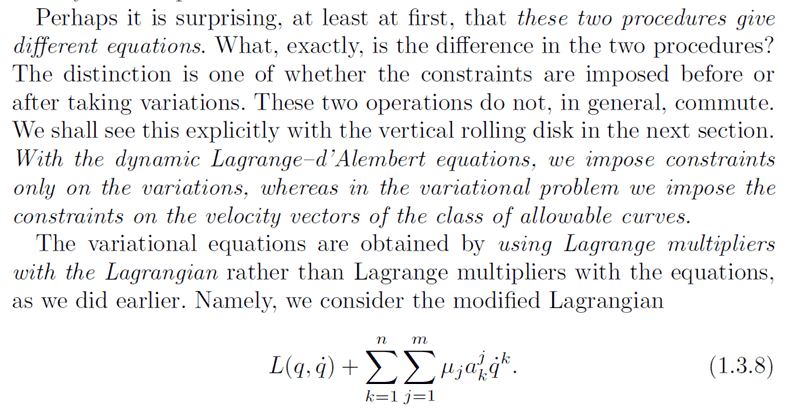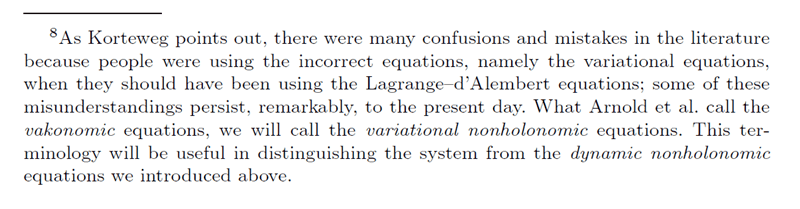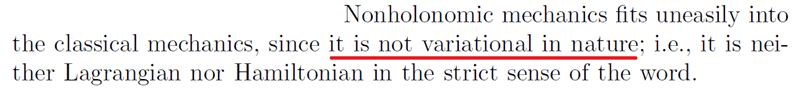# Is Hamilton's principle an empirical statement?

The principle of the least action, that the particle will take the path of least Lagrangian, here given as T-U, is Hamilton's principle in classical mechanics. I am wondering if this is just an empirical, experimental observation that is not mathematically driven from elsewhere, just like Newton's three laws.

Thank you for the help.

Oh the Lagrangian does not necessarily be T-U

wrobel
Hamilton's principle is derived from Newton's laws + hypothesis on constrains they must be ideal and holonomic

Last edited:
vanhees71
Gold Member
2021 Award
As discussed recently, Hamilton's principle can also be used to derive the correct equations of motion for the case of nonholonomic constraints. The equations are identical with the ones found using d'Alembert's principle. The distinction from holonomic constraints is that the nonholonomic constraints are constraints on the allowed trajectories in the variational principle, i.e., on the variations ##\delta q## and not on the configuration space of the system.

wrobel
Let's clarify it by a simplest example.

Assume we have a Lagrangian

$$L=\frac{1}{2}g_{ij}\dot x^i\dot x^j,\quad i,j=1,\ldots, m.$$ The coefficients ##g_{ij}## form a Riemann metric; for simplicity we assume that all ##g_{ij}## are constants.

Consider a variational problem of finding extremums of a functional

$$x(\cdot)\mapsto \int_{t_1}^{t_2}Ldt$$ in the class of smooth functions ##x(t)=(x^1,\ldots,x^m)(t)## such that ##x(t_i),\quad i=1,2## are fixed and $$a_i(x)\dot x^i=0,\quad t\in[t_1,t_2].\qquad (*)$$

From Calculus of Variations [see for example Bruce van Brunt: The Calculus of Variations. Springer 2004] it is known that the extremum to this problem is a solution to the Lagrange equations with the following Lagrangian ##\mathcal L(x,\dot x)=L-\lambda a_k\dot x^k##. Simultaneously the multiplier ##\lambda=\lambda(t)## is chosen to fulfill equations (*).

The system with Lagrangian ##\mathcal L## is written as follows

$$g_{si}\ddot x^i-\dot\lambda a_s-\lambda\dot x^p\Big(\frac{\partial a_s}{\partial x^p}-\frac{\partial a_p}{\partial x^s}\Big)=0. \qquad (**)$$

To express ##\dot\lambda## let us differentiate formula (*) in ##t##:

$$\frac{\partial a_k}{\partial x^n}\dot x^k\dot x^n+a_k\ddot x^k=0.$$

Substituting here ##\ddot x## from (**) we finally yield

$$\dot\lambda=-\frac{1}{g^{ij}a_ia_j}\Big(\frac{\partial a_i}{\partial x^n}\dot x^n\dot x^i+\lambda a_i\dot x^sg^{ik}\Big(\frac{\partial a_k}{\partial x^s}-\frac{\partial a_s}{\partial x^k}\Big)\Big) .\qquad (***)$$

Generally equations (**)-(***) describe the vaconomic mechanics of system with Lagrangian ##L##. To obtain a solution to this system we must impose the initial conditions: ##x(0),\dot x(0),\lambda(0).## For the same ##x(0),\dot x(0)## we can take different ##\lambda(0)## and obtain different solutions ##x(t)##. This means that from the "classical" standpoint when we trace only the function ##x(t)##, system (**)-(***) does not possesses the uniqueness property. Thus in general it can not be a system of classical mechanics.

We point just a simplest case when system (**)-(***) turns to be a system of classical mechanics. Assume that $$\frac{\partial a_s}{\partial x^p}-\frac{\partial a_p}{\partial x^s}=0.\qquad (!)$$ Then substituting ##\dot\lambda## from (***) to (**) we get

$$g_{si}\ddot x^i+\frac{a_s}{g^{ij}a_ia_j}\frac{\partial a_i}{\partial x^n}\dot x^n\dot x^i=0.$$

It is not hard to show that these equations describe the classical Lagrangian system with Lagrangian ##L## and ideal constraints (*).

Introduce a differential form ##\omega=a_idx^i##. Then the condition (!) implies that locally there exists a function ##f=f(x)## such that ##df=\omega## i.e. the constraint (*) is integrable and our system is holonomic. In this case the described above variational problem tuns to be the Hamilton principle. Generally, necessary and sufficient condition of integrability of constraint (*) is as follows ##\omega\wedge d\omega=0##. In case of nonholonomic constraint the formulated above variational problem does not give equations of classical mechanics.

Thus it important not to mix up Hamilton's variational principle and the Lagrange-d'Alembert principle

Last edited:
vanhees71
Gold Member
2021 Award
We should not repeat this superfluous discussion again. The vaskonomic dynamics is flawed and not the correct variational principle. Instead one has implement the non-holonomic constraints as constraints on the variations around the "true" trajectory, i.e. in the sense of virtual displacements as in the d'Alembert principle. This means you have to minimize
$$A[q]=\int_{t_1}^{t_2} \mathrm{d} t L(q,\dot{q},t)$$
for all variations around the stationary point ##q(t)## which are subject to the constraints
$$a_{ik}(q,t) \delta q^{k}=0.$$
To implement them you take the variation of ##A## and add the usual Lagrange-multiplier terms:
$$\delta A[q] = \int_{t_1}^{t_2} \mathrm{d} t \delta q^{k} \left [\frac{\partial L}{\partial q^k}-\frac{\mathrm{d}}{\mathrm{d} t} \frac{\partial L}{\partial \dot{q}^k} - \lambda^i a_{ik} \right] \stackrel{!}{=}0,$$
which leads to the equations of motion
$$\frac{\partial L}{\partial q^k}-\frac{\mathrm{d}}{\mathrm{d} t} \frac{\partial L}{\partial \dot{q}^k}=\lambda^i a_{ik}.$$
Now, for the "true trajectory" you can write the non-holonomic contraints as
$$a_{ik} \dot{q}^k=0.$$
Note that the vaskonomic dynamics and the correct d'Alembert dynamics coincide if and only if the an-holonomic constraints are integrable, i.e., can be implemented as holonomic constraints.

wrobel
We should not repeat this superfluous discussion again
ok, then cite please a book which contains such a statement:
This means you have to minimize
A[q]=∫t2t1dtL(q,˙q,t)​
A[q]=\int_{t_1}^{t_2} \mathrm{d} t L(q,\dot{q},t)
for all variations around the stationary point q(t)q(t) which are subject to the constraints
aik(q,t)δqk=0.​
and this statement is called the Hamilton variational principle (for nonholonomic constraints, you know).

It would be great if you cut the corresponding text from that book and post the scan here

vanhees71
Gold Member
2021 Award
But, I have derived the nonholonomic equations of motion from the variational principle in the way shown in my previous posting. So why are you saying that's wrong? It's in any good textbook on analytical mechanics (Landau&Lifshitz vol. 1, Sect. 38).

wrobel
But, I have derived the nonholonomic equations of motion from the variational principle in the way shown in my previous posting. So why are you saying that's wrong?
Because this is not a variational problem. Variational problem is a problem about extremums of a functional which is defined on some manifold with constraints. When you solve a variational problem you can not choose variations as you want. The constraints determine the space of variations.
So the phrase:
This means you have to minimize
A[q]=∫t2t1dtL(q,˙q,t)​
A[q]=\int_{t_1}^{t_2} \mathrm{d} t L(q,\dot{q},t)
for all variations around the stationary point q(t)q(t) which are subject to the constraints
aik(q,t)δqk=0.​
is meaningless in the mathematical sense. The constraints ##a_i\dot x^i=0## imply the following space of variations ##\{\delta x^j\mid a_i\frac{d}{dt}(\delta x^i)+\frac{\partial a_i}{\partial x^k}\dot x^i\delta x^k=0\}##. This corresponds to the vaconomic mechanics. But the Largange-D'Alembert equations do not correspond to conditional extremum of any functional in nonholonomic case.

And please never tell to specialists in dynamical systems that Landau&Lifshitz vol. 1 is a good book they will die laughing

vanhees71
Gold Member
2021 Award
Because this is not a variational problem. Variational problem is a problem about extremums of a functional which is defined on some manifold with constraints. When you solve a variational problem you can not choose variations as you want. The constraints determine the space of variations.
So the phrase:

is meaningless in the mathematical sense. The constraints ##a_i\dot x^i=0## imply the following space of variations ##\{\delta x^j\mid a_i\frac{d}{dt}(\delta x^i)+\frac{\partial a_i}{\partial x^k}\dot x^k\delta x^i=0\}##. This corresponds to the vaconomic mechanics. But the Largange-D'Alembert equations do not correspond to conditional extremum of any functional in nonholonomic case.

And please never tell to specialists in dynamical systems that Landau&Lifshitz vol. 1 is a good book they will die laughing
I don't bother, how you call what I did. I find this very straight forward, and I never understood, why non-holnomic constraints are more complicated than holonomic ones. Again the logic is the following. One defines the action functional as usual
$$A[q]=\int_{t_1}^{t_2} \mathrm{d} t L(q,\dot{q},t).$$
Then one defines the (in general nonholonomic) constraints as constraints on the allowed variations
$$a_{ik}(q,t) \delta q^k=0.$$
These have to be included by the introduction of Lagrange multipliers in the usual way, leading to
$$\delta A[q] = \int_{t_1}^{t_2} \mathrm{d} t [\delta L-\lambda^{i} a_{ik} \delta q^k] \stackrel{!}{=}0.$$
Thanks to the Lagrange multipliers now we can vary the ##\delta q^k## independently and get the usual equations of motion as from d'Alembert's principle,
$$\frac{\partial L}{\partial q^k}-\frac{\mathrm{d}}{\mathrm{d} t} \frac{\partial L}{\dot{q}^k}=\lambda^{i} a_{ik}.$$
That's, how it's derived in Landau&Lifshitz vol. I, Section 38 too, as I've just checked.

wrobel
I apologize for the harshness

vanhees71
Gold Member
2021 Award
No problem; physics discussions sometimes get excited :-)).

wrobel
O, it seems I have found proper words.
Logic of variational problem is as follows. (little bit informally) What is ##\delta A##? To calculate it we do the following procedure:
##\delta A=\frac{d}{ds}\Big|_{s=0}\int_{t_1}^{t_2}L\Big(x+s\delta x,\frac{d}{dt}\Big( x+s\delta x\Big)\Big)dt.##That is we differentiate the functional ##A## on the set ##\{ x+s\delta x\}## with ##x(t)## fixed. To determine the space of variations ##\delta x## we must do the same for the equation of constraint:
$$a_i(x)\dot x^i=0\Longrightarrow\frac{d}{ds}\Big|_{s=0}\Big[a_i(x+s\delta x)\frac{d}{dt}\Big(x^i+s\delta x^i\Big)\Big]=0.$$
It is exactly the same as when we look for conditional minimum for example for the function ##f(u,v)=u-v## under constraint ##u^2+v^2=1##.

Last edited:
vanhees71
Gold Member
2021 Award
Hm, but aren't you then lead back to the vakonomic dynamics again. The point is that in your ##\delta A## the ##\delta x^i## are not independent but subject to the non-holonomic constraint
$$a_i \delta x^i=0.$$
$$\delta x^k \partial_k a_i(x) \dot{x}^i+a_i \frac{\mathrm{d}}{\mathrm{d} t} \delta x^i=0.$$
This is something different than the correct d'Alembertian virtual displacement constraint given above.

wrobel
Hm, but aren't you then lead back to the vakonomic dynamics again
Sure! it is vaconomic again
δxk∂kai(x)˙xi+aiddtδxi=0.​
Sure, but this equation must be imposed if we want to minimize the functional ##A## on the space of functions ##\{x(t)\mid a_i(x(t))\dot x^i=0\}##. It is obviously not the same as ##a_i\delta x^i=0##. It is the main thing I am trying to say: the Lagrange-d'Alembert equations are not generated by the problem of minimization.

wrobel
The problem of minimization of the functional ##A## on ##\{x(t)\mid a_i(x(t))\dot x^i=0\}## is exactly the vaconomic problem

vanhees71
Gold Member
2021 Award
Yes, and IT IS WRONG! The constraint must be implemented as I wrote to get the correct equations of motion as from the d'Alembert principle. This is really standard textbook stuff!

wrobel
Yes, and IT IS WRONG!
what exactly is wrong? Just cite my phrase

vanhees71
Gold Member
2021 Award
To assume that you can derive the correct equations by treating it as you say, because it leads to vakonomic dynamics. You have to impose the conditions in the sense I wrote and as is written in almost all textbooks I know. The only exception is Goldstein, and he's not even discussing that he gets the wrong vakonomic dynamics!

wrobel
To assume that you can derive the correct equations by treating it as you say
I have never assumed that. Please read cearfully what I have written

vanhees71
Gold Member
2021 Award
I obviously don't understand your problem. What's in your opinion wrong with the really simple standard treatment, and why do you insist on the wrong variational principle leading to vakonomic dynamics?

wrobel
I just stress two things.

1) The problem of minimization of the functional ##A## on the space ##\{x(t)\mid a_i(x(t))\dot x^i=0\}## leads to the vaconomic equations. It is a fact from variational calculus, I have already cited the textbook.
2) In general the Lagrange-D'Alembert equations do not follow from the variational principle formulated in 1).

vanhees71
Gold Member
2021 Award
Both statements are wrong. As I've shown repeatedly in this thread you only have to impose the non-holonomic constraints correctly as a constraint on the (instantaneous!) variations and not in the way of your item (1). I don't understand, how one came to the idea to use (1) as a constraint in the first place since we know since d'Alembert how to do it right.

So I guess, we have to leave it at that and agree to disagree.

wrobel
I don't understand, how one came to the idea to use (1)
it is an interesting storyand from the Preface of Bloch's book:vanhees71